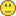New to QlikView

Discussion board where members can get started with QlikView.

Announcements
QlikWorld 2020: Join us May 11 - 14, 2020 in Phoenix, AZ. Register early and save \$400. Learn More
Honored Contributor III

Calculate opening balance

Hi,

I am trying to show opening balance. i am having month field

TBAL_CAL_MONTH

0

1

2

3

4

5

6

7

8

9

10

11

12

if i select 7th month it should show the value of 0-6 th month as opening balance

i tried below code but no luck

sum({<TBAL_CAL_MONTH={">=\$(=MonthsStart(Date(Max(TBAL_CAL_MONTH))))<=\$(=Max(Date(TBAL_CAL_MONTH)))"}>}TBAL_MTD_DR_LC_1-TBAL_MTD_CR_LC_1)

plz suggest

thanks

27 Replies
Honored Contributor III

Re: Calculate opening balance

is this Rolling month?

plz suggest some advice

Honored Contributor III

Re: Calculate opening balance

any solutions?Valued Contributor II

Re: Calculate opening balance

hi der

try this

sum({<TBAL_CAL_MONTH={">=\$(=min({1}TBAL_CAL_MONTH))<\$(=max(TBAL_CAL_MONTH))"}>}TBAL_MTD_DR_LC_1-TBAL_MTD_CR_LC_1)

Honored Contributor III

Re: Calculate opening balance

Hi

thanks for replying but it showing zeros onlyNot applicable

Re: Calculate opening balance

try this:

sum({<TBAL_CAL_MONTH={"<\$(=Max(TBAL_CAL_MONTH))"}>}TBAL_MTD_DR_LC_1-TBAL_MTD_CR_LC_1)

is there a year field?

Valued Contributor II

Re: Calculate opening balance

post sample dataPartner

Re: Calculate opening balance

RangeSum(Above(sum( {<TBAL_CAL_MONTH={"<\$(=Max(TBAL_CAL_MONTH))"}>}TBAL_MTD_DR_LC_1-TBAL_MTD_CR_LC_1),0,RowNo()))

Honored Contributor III

Re: Calculate opening balance

here is the sample app

Honored Contributor III

Re: Calculate opening balance

Hi Simson,

Thanks for reply but the result is not correct